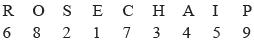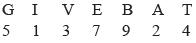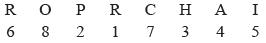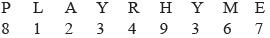Courses

# Olympiad Test: Coding Decoding - 3

## 20 Questions MCQ Test National Cyber Olympiad Class 8 | Olympiad Test: Coding Decoding - 3

Description
This mock test of Olympiad Test: Coding Decoding - 3 for Class 8 helps you for every Class 8 entrance exam. This contains 20 Multiple Choice Questions for Class 8 Olympiad Test: Coding Decoding - 3 (mcq) to study with solutions a complete question bank. The solved questions answers in this Olympiad Test: Coding Decoding - 3 quiz give you a good mix of easy questions and tough questions. Class 8 students definitely take this Olympiad Test: Coding Decoding - 3 exercise for a better result in the exam. You can find other Olympiad Test: Coding Decoding - 3 extra questions, long questions & short questions for Class 8 on EduRev as well by searching above.
QUESTION: 1

### If in a certain code YELLOW is written as XFKMNX, how is country coded ?

Solution:

In the code, the letters in the odd place are one before those in the even places are one ahead than the corresponding letter in the word.

QUESTION: 2

### In a certain language MECHANICS is coded as HCEMASCIN, how is POSTER coded in that code?

Solution:

In the code the first four and the last four letters are reversed in order.

QUESTION: 3

### In a certain code MOUNTAIN is written as OMNUATNI, how is READER written in that code?

Solution:

The code is obtained by interchanging the positions of the first and second letters, third & fourth letters and soon

QUESTION: 4

In a certain code language TELEPHONE is written as ENOHPELET, how is ALIGATOR written in that code?

Solution:

The letters of the given word and written in the reverse order in the code.

QUESTION: 5

It PLANE is coded as OKZMD in a certain language, how will TRAIN be coded?

Solution:

The letters preceding each letter of the given word in the alphabets are taken as the subsequent letter of the code.

QUESTION: 6

In a certain language champion  is coded as HCMAIPNO, how is NEAGTIVE coded in that code?

Solution:

In the code, each of the two letters are reversed in arrangement.

QUESTION: 7

In a certain language PEARL is coded as SHDUO, how is COVET coded in that code?

Solution:

Each letter in the code is two ahead of the corresponding letter in the world.

QUESTION: 8

IF table is coded GZYOV, how is JUICE coded?

Solution:

It in the word, a letter is nth alphabet from the beginning, then in the code corresponding letter is the nth alphabet from the end.

QUESTION: 9

In a certain language BLEMISH is coded as AODPHVG, how is CHAPTER coded in that code?

Solution:

In the code, the letters at odd places are one before and those at odd places are there places ahead of the corresponding letters in the word.

QUESTION: 10

In a certain code , GOODNESS is coded as HNPCODTR, how is GREATNESS coded in that code?

Solution:

Letters at odd place in the coded word are one letter at even places are one letter behind of the letters in the basic word as their positions in the alphabets.

QUESTION: 11

It porter is written as QNSSFQ, them BRIGHT would be coded as

Solution:

Letters at the odd places in the coded word the basic word, and letter at even places in the coded word are one letter back of the respective letters as their position in the alphabet.

QUESTION: 12

In a certain language, DIUGNAL is code for LANGUID, which word would be coded as ELKCAHS?

Solution:

In the code, the letters of words are put in a reverse order.

QUESTION: 13

In a certain code covet is written as FRYHW, which word would be written as SHDUO?

Solution:

Each letter of the word is three steps behind the corresponding letter of the code.

QUESTION: 14

If BAFFLE is AATUCX, then DANGER is to

Solution:

Codes for the letters have been used in forward direction with a gap of 0, 1, 2, 3, 4, and 5 positions, regarding position of their codes in the given table.

QUESTION: 15

If MAIDEN is to 44x2b6, then FIBRES is to:

Solution:

For second & fifth letters, codes have been used from just below the letters, for the first & fourth letter, code have been used from one position back, and for third & sixth letter codes have been used are position ahead as their position in the table.

QUESTION: 16

In a MAIDEN is to 45v4zb, then DANGER is to:

Solution:

In the word MAIDEN code for MA, ID and EN have been Exchanged.

QUESTION: 17

It Rose is coded as 6821, chair is coded as 73456 and PREACH is coded as 961473, what will be the code for SERCH?

Solution:

The alphabet are shown i.e S as 2, E as 1, as 4, R as 6, C as 7, and H as 3. So, search is coded as 214673.QUESTION: 18

If GIVE is coded as 5137 and BAT is coded as 924, how is GATE coded?

Solution:

The alphabet are coded as shown i.e G as 5, A as 2, T as 4, and E as 7. So GATE is coded as 5247QUESTION: 19

It coded as 6821 and CHAIR is coded as 73456 then what will be the code for CREPE?

Solution:

The alphabet are coded as shown i.e C as 7, R as 6, A as 4, P as 2 and E as I. Thus, CRAPE is coded as 76421QUESTION: 20

If PLAY is coded as 8123 and RHYME is coded as 49367, how is MALE coded?

Solution:

The alphabet are coded as shown i.e M as 6, A as 2, L as 1 and E as 7. So , male is coded as 6217